## Variation of Argument

Letdenote the change in argument of a functionaround a closed loop. Also letdenote the number of Roots ofinanddenote the number of Poles ofin. Then(1)

To findin a given region, breakinto paths and findfor each path. On a circular Arc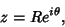(2)

letbe a Polynomialof degree. Then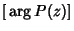(3)

Plugging in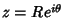gives(4)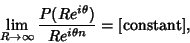(5)

so(6)

and(7)

For a Real segment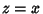,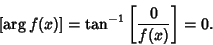(8)

For an Imaginary segment,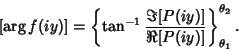(9)

Note that the Argument must change continuously, so jumps'' occur across inverse tangent asymptotes.